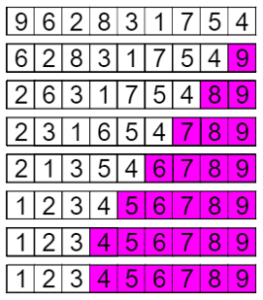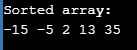AWS Global Infrastructure

### Programming & Frameworks

Topics Covered
• C Programming and Data Structures (16 Blogs)
• Comprehensive Java Course (4 Blogs)
• Java/J2EE and SOA (346 Blogs)
• Spring Framework (8 Blogs)
SEE MORE

# How to Implement Bubble Sort in C with CodeBubble sort in C is a simple sorting algorithm which repeatedly compares the adjacent elements of the given array & swaps them if they are in the wrong order. You might be wondering about the name Bubble Sort. Following are the Pointers Covered in this article:

## What is a Bubble Sort in C?

The sorting technique is called so because the algorithm acts like a bubble, the lighter elements come up and heavier elements settle down. Bubble Sort algorithm sorts the list in passes. Now, to sort a list with n elements Bubble sort requires n-1 passes. To make it clearer, let’s understand this step by step.## Algorithm of Bubble Sort

• Pass 1
• X & X are compared, and swapped if X > X
• X & X are compared, and swapped if X > X
• X & X are compared, and swapped if X > X and so on…
• At the end of pass 1, the largest element of the list is placed at the highest index of the list.
• Pass 2:
• X & X are compared, and swapped if X > X
• X & X are compared, and swapped if X > X
• X & X are compared, and swapped if X > X and so on…
• At the end of Pass 2 the second largest element of the list is placed at the second-highest index of the list.
• Pass n-1:
• X & X are compared, and swapped if X > X
• X & X are compared, and swapped if X > X
• X & X are compared, and swapped if X > X and so on…
• At the end of this pass. The smallest element of the list is placed at the first index of the list.

## Example of Bubble Sort in C

Array: -5, 35, 2, 13, -15

Pass 1

• -5, 35, 2, 13, -15) –> ( -5, 35, 2, 13, -15), Here, algorithm compares the first two elements.
• ( -5, 35, 2, 13, -15) –>  (-5, 2, 35, 13, -15), Swap since 35 > 2
• ( -5, 2, 35, 13, -15) –>  (-5, 2, 13, 35, -15), Swap since 35 > 13
• ( -5, 2, 13, 35, -15) –>  (-5, 2, 13, -15, 35), Swap since 35 > -15

The last element is the largest element.

Pass 2

• (-5, 2, 13, -15, 35) –>  (-5, 2, 13, -15, 35)
• (-5, 2, 13, 35, -15) –>  (-5, 2, 13, -15, 35)
• (-5, 2, 13, -15, 35) –>  (-5, 2, -15, 13, 35), Swap since 13 > -15

The second last element is the second largest element.

Pass 3

• (-5, 2, -15, 13, 35) –>  (-5, 2, -15, 13, 35)
• (-5, 2, -15, 13, 35) –>  (-5, -15, 2, 13, 35), Swap since 2 > -15

The third last element is the third largest element.

Pass 4

• (-5, -15, 2, 13, 35) –>  (-15, -5, 2, 13, 35) , Swap since -5 > -15

Eventually, the first is the smallest & 2nd is the second smallest element in the array.  So, in this case, four passes were required to sort an array of 5 elements.

Before looking at the algorithm in detail, let’s look at the time complexity of the Bubble Sort in C algorithm.

## The complexity of Bubble Sort

• Worst Case Complexity: O(n2)
• Best Case Complexity: O(n2)
• Average Case Complexity: O(n)

Now let us quickly look at the algorithm, so that moving ahead we can write the Bubble sort algorithm in C.

## Bubble Sort Function

```void bubbleSort(int array[], int n)
{
int i, j;
//Pass in Bubble Sort
for (i = 0; i < n-1; i++)

/* Comparing the two adjacent elements & swapping if elements are not at the correct position */
for (j = 0; j < n-i-1; j++) if (array[j] > array[j+1])
swap(&array[j], &array[j+1]);
}
```

## Bubble Sort in C Program

```#include <stdio.h>

// Function to swap elements
void swap(int *a, int *b)
{
int temp = *a;
*a = *b;
*b = temp;
}

// bubble sort function
void bubbleSort(int array[], int n)
{
int i, j;
for (i = 0; i < n-1; i++)

for (j = 0; j < n-i-1; j++) if (array[j] > array[j+1])
swap(&array[j], &array[j+1]);
}

// Function to print the elements of an array
void printArray(int array[], int size)
{
int i;
for (i=0; i < size; i++)
printf("%d ", array[i]);
printf("n");
}

// Main Function
int main()
{
int array[] = {-5, 35, 2, 13, -15};
int size = sizeof(array)/sizeof(array);
bubbleSort(array, size);
printf("Sorted array: n");
printArray(array, size);
return 0;
}```Now after executing the above C program you would have understood how Bubble Sort works & how to implement it in C language. I hope this blog is informative and added value to you.

Check out the Java training by Edureka, a trusted online learning company with a network of more than 250,000 satisfied learners spread across the globe. Edureka’s Java J2EE and SOA training and certification course is designed for students and professionals who want to be a Java Developer. The course is designed to give you a head start into Java programming and train you for both core and advanced Java concepts along with various Java frameworks like Hibernate & Spring.

Got a question for us? Please mention it in the comments section of this Bubble Sort in C article and we will get back to you as soon as possible.REGISTER FOR FREE WEBINARThank you for registering Join Edureka Meetup community for 100+ Free Webinars each month